Orginal Article

# 二维稳定流形的自适应推进算法

Yuan Guoqiang*, Li Yinghui

School of Aeronautics and Astronautics Engineering, Air Force Engineering University,Xi’an 710038, China

Abstract

Keywords： stable manifolds ; unstable manifolds ; invariant manifolds ; adaptive front advancing algorithm ; dynamical system

0

Yuan Guoqiang*, Li Yinghui. ADAPTIVE FRONT ADVANCING ALGORITHM FOR COMPUTING TWO-DIMENSIONAL STABLE MANIFOLDS[J]. Chinese Journal of Theoretical and Applied Mechanics, 2018, 50(2): 405-414 https://doi.org/10.6052/0459-1879-17-353

## 1 稳定和不稳定流形

$x=f(x)(1)$

$$\left. \bal W^{\rm s} ({\pmb x}_0 ) = \{{\pmb x} \in {\bf R} ^n\vert \mathop {\lim}\limits_{t \to \infty } \phi (t,{\pmb x}_0 ) = {\pmb x}_0 \} \\ W^{\rm u} ({\pmb x}_0 ) = \{{\pmb x} \in {\bf R}^n\vert \mathop {\lim}\limits_{t \to \infty } \phi ( - t,{\pmb x}_0 ) = {\pmb x}_0 \} \end{array} \right\} (2)$$

## 2 二维稳定流形自适应推进算法

### 2.1 初始邻域和初始备选网格

$$\left.\!\!\begin{array}{l} {\pmb q}_1 = {\pmb e}_1 / \left\| {{\pmb e}_1 } \right\| \\\\ {\pmb q}_2 = {\pmb e}_2 - \dfrac{({\pmb e}_2 ,{\pmb q}_1 )}{({\pmb q}_1 ,{\pmb q}_1 )}{\pmb q}_1 \Bigg / \left\| {{\pmb e}_2 - \dfrac{({\pmb e}_2 ,{\pmb q}_1 )}{({\pmb q}_1 ,{\pmb q}_1 )}{\pmb q}_1 } \right\| \end{array}\!\! \right\} (3)$$

$p(i)=[q1sin(2i/N)+q2cos(2i/N)]R+x0(4)$

$e1,e2$为复向量,系统的稳定特征子空间无法由 $e1,e2$直接张成,但可以利用距离平衡点 $x0$较近的一段轨道构造平行于稳定特征子空间的两个实相量. 记 $e1,2=u±iv$,其对应的特征值为 $α±iβ$.图1所示,由点 $x=x0+Ru/u$出发,对系统(1)按时间反向积分,积分时间 $Δt=/(2β)$,得到点 $xq=ϕ(Δt,x)$,继续积分得到点 $xh=ϕ(2Δt,x)$. 于是可以得到平行于系统稳定特征子空间的向量 $xqx$, $xqxh$.$xqx$, $xqxh$按式(3)正交单位化,即可由式(4)生成初始邻域的前沿点.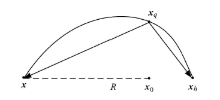Fig. 1   Schematic of determination of stable eigenspaces based on trajectory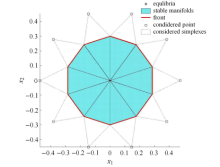Fig. 2   Schematic of initial neighborhood and initial considered mesh

### 2.2 相切条件

$g(x1,x2)-x3=0(5)$

$Ws(x0)$与曲面 $g(x1,x2)-x3=0$$x$处相切,既有如式(6)的偏微分方程 

$$f(x_1 ,x_2 ,g(x_1 ,x_2 )) \left[\!\! \begin{array}{ccc} {\dfrac{\partial g}{\partial x_1 }} & {\dfrac{\partial g}{\partial x_2}} & { - 1}\end{array} \!\! \right]^{\rm T}=0 (6)$$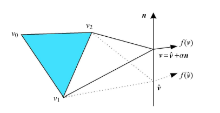Fig. 3   Geometric explanation of the tangent condition

$${\pmb n}^{\rm T}f(\hat{\pmb v} + \alpha {\pmb n}) = 0 (7)$$

### 2.3 单元尺寸自适应

$$\varDelta _{AB\hat {v}} = \dfrac{2h_C + \varepsilon }{ h_A + h_B + \varepsilon}\varDelta _{ABC} (8)$$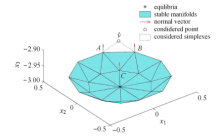Fig. 4   Adaptability of mesh simplex size

### 2.4 自适应推进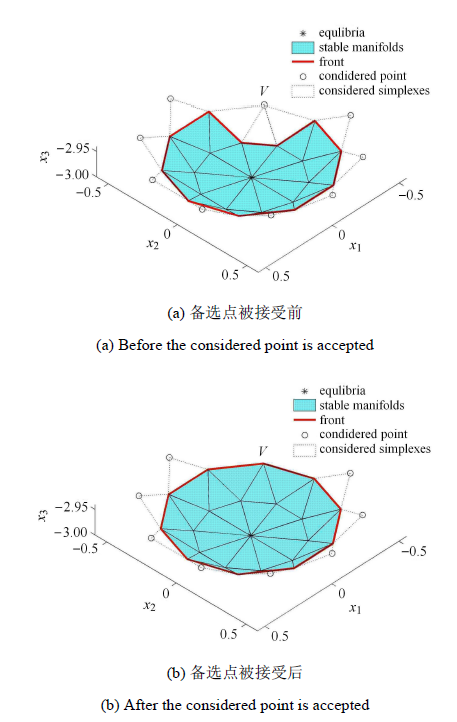## 5 接受备选点与更新前沿Fig. 5   Accepting considered points and updating front

$vkvl$为新接受三角形 $vkvlvi$的一条边,且 $vkvl$位于稳定流形网格的前沿, $vkvl=L$. 现考虑为 $vkvl$扩展出新的备选点,考察所有与 $vkvl$相邻的边(含备选三角形的边). 记 $vjvk$$vlvm$为与 $vkvl$相邻且处于现有网格(含备选网格)最外围的边,则 $vjvk$$vlvm$为前沿边或备选三角形的边. 不妨设 $vlvm$为前沿边,则 $vm$为稳定流形上的已知点,且 $vlvm$没有对应的备选点. 此时现有网格的最外围是由前沿边和备选三角形边组成的空间多边形, 并称最外围的边为外围边. 记 $γ1=∠vjvkvl$$vjvk$$vkvl$所构成的外角, $γ2=∠vkvlvm$$vkvl$$vkvm$所构成的外角, $γ=min(γ1,γ2)$. 根据 $γ$的大小,分以下两种情形产生新的备选点.

$$L_1 = \left\{ \!\! \begin{array}{ll} {2L\,,} & {L \leqslant 0.5\varDelta _{v_{\rm k} v_{\rm l} v} } \\\\ {\varDelta _{v_{\rm k} v_{\rm l} v} \,,} & {0.5\varDelta _{v_{\rm k} v_{\rm l} \end{array} \!\! \right. (9)$$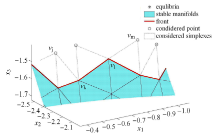Fig. 6   Sketch map of new alternative triangle generation when $γ<π/2$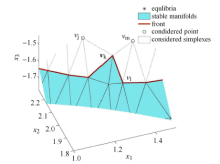Fig. 7   Sketch map of new alternative triangle generation when $β=8/3$

## 3 算例应用

### 3.1 洛伦兹流形

$$\left. \begin{array}{l} {\dot {x}_1 = \varsigma (x_2 - x_1 )} \\ {\dot {x}_2 = \rho x_1 - x_2 - x_1 x_3 } \\ {\dot {x}_3 = - \beta x_3 + x_1 x_2 } \end{array} \right\} (10)$$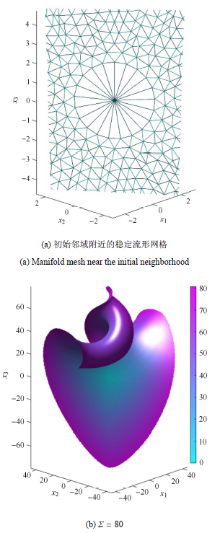Fig. 8   The computational process of Lorentz manifolds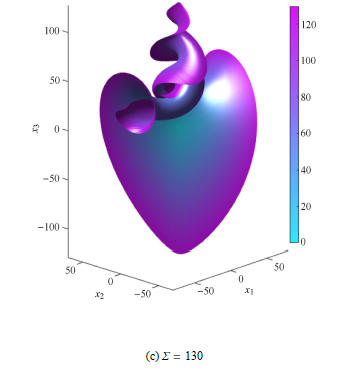Fig. 8-1   The computational process of Lorentz manifolds (continued)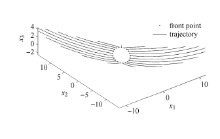Fig. 9   The difference of the evolution velocity of vector field in different directions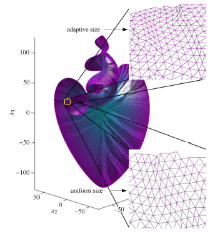Fig. 10   Mesh comparison between the proposed algorithm and the PDE approach

### 3.2 类球面流形

$$\left. \begin{array}{l} {\dot {x}_1 = (p(r,x_3 ) + x_3 )x_1 } \[2mm] {\dot {x}_2 = (p(r,x_3 ) + x_3 )x_2 } \[2mm] {\dot {x}_3 = p(r,x_3 )x_3 - x_1^2 - x_2^2 }\end{array} \right\} (11)$$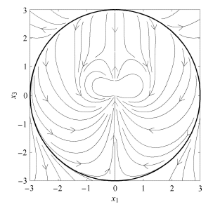Fig. 11   The phase portrait in the {Invalid MML} plane of the system (11)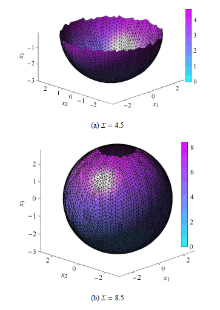Fig. 12   Computational process of sphere-like manifolds

## 4 结 论

(1)该算法对流形曲率具有全局自适应性,能根据稳定流形的曲率自适应地调整网格计算单元的尺寸,因而能持续有效的计算全局稳定流形.

(2)该算法中备选点的生成规则是由稳定流形的几何特征决定的,能充分利用流形曲率等几何特征,因此流形网格的扩展不受系统向量场在各方向上演化速度差异的影响.

The authors have declared that no competing interests exist.

## 参考文献 原文顺序 文献年度倒序 文中引用次数倒序 被引期刊影响因子

  Guckenheimer J, Krauskopf B, Osinga HM, et al.Invariant manifolds and global bifurcations.Chaos: An Interdisciplinary Journal of Nonlinear Science, 2015, 25(9): 097604  贾蒙. 高维非线性映射系统的不稳定流形计算方法研究. 振动与冲击, 2017, 36(17): 262-266 (Jia Meng.Computation method for unstable manifold of hénon map.Journal of Vibration and Shock, 2017, 36(17): 262-266 (in Chinese))  郑丹丹, 罗建军, 张仁勇等. 基于混合Lie算子辛算法的不变流形计算. 力学学报, 2017, 49(5): 1126-1134 (Zheng Dandan, Luo Jianjun, Zheng Renyong, et al.Computation of invariant manifold based on symplectic algorithm of mixed Lie operator.Chinese Journal of Theoretical and Applied Mechanics, 2017, 49(5): 1126-1134 (in Chinese))  Qu L, Li YH, Xu HJ, et al.Aircraft nonlinear stability analysis and multidimensional stability region estimation under icing conditions.Chinese Journal of Aeronautics, 2017, 30(3): 976-982  贾建华, 吕敬, 王琪. 带脉冲的三维引力辅助变轨研究. 力学学报, 2016, 48(2): 437-446 (Jia Jianhua, Lü Jing, Wang Qi.Powered gravity assist in three dimensions.Chinese Journal of Theoretical and Applied Mechanics, 2016, 48(2): 437-446 (in Chinese))  李渊, 邓子辰, 叶学华等. 基于辛理论的载流碳纳米管能带分析. 力学学报, 2016, 48(1): 135-139 (Li Yuan, Deng Zichen, Ye Xuehua, et al.Analysing the wave scattering in single-walled carbon nanotube conveying fluid based on the symplectic theory.Chinese Journal of Theoretical and Applied Mechanics, 2016, 48(1): 135-139 (in Chinese))  钱霙婧, 翟冠峤, 张伟. 基于多项式约束的三角平动点平面周期轨道设计方法. 力学学报, 2017, 49(1): 154-164 (Qian Yingjing, Zhai Guanqiao, ZhangWei. Planar periodic orbit construction around the triangular libration points based on polynomial constraints.Chinese Journal of Theoretical and Applied Mechanics, 2017, 49(1): 154-164 (in Chinese))  Wang T, Chiang HD.On the number of unstable equilibrium points on spatially-periodic stability boundary.IEEE Transactions on Automatic Control, 2016, 61(9): 2553-2558  袁国强, 李颖晖. 积冰对飞机本体纵向非线性动力学稳定域的影响. 西安交通大学学报, 2017, 51(9): 153-158 (Yuan Guoqiang, Li Yinghui.Effect of ice accretion on aircraft longitudinal nonlinear dynamical stability region.Journal of Xi’an Jiaotong University, 2017, 51(9): 153-158 (in Chinese))  Chiang HD, Wang T.On the number and types of unstable equilibria in nonlinear dynamical systems with uniformly-bounded stability regions.IEEE Transactions on Automatic Control, 2016, 61(2): 485-490  Alberto LFC, Chiang HD.Characterization of stability region for general autonomous nonlinear dynamical systems.IEEE Transactions on Automatic Control, 2012, 57(6): 1564-1569  Krauskopf B, Osinga HM, Doedel EJ, et al.A survey of methods for computing (un)stable manifolds of vector fields.International Journal of Bifurcation and Chaos, 2005, 15(3): 763-791  Kuznetsov YA.Elements of Applied Bifurcation Theory. 2nd ed. NY: Springer Verlag, 1998  Guckenheimer PJ, Holmes. Nonlinear oscillations, dynamical systems and bifurcations of vector fields. 2nd ed. NY: Springer-Verlag, 1986  Johnson ME, Jolly MS, Kevrekidis IG.Two-dimensional invariant manifolds and global bifurcations: Some approximation and visualization studies.Numerical Algorithms, 1997, 14(1): 125-140  Krauskopf B, Osinga HM.Computing geodesic level sets on global (un)stable manifolds of vector fields.SIAM Journal on Applied Dynamical Systems, 2003, 2(4): 546-569  Guckenheimer J, Worfolk P.Dynamical systems: Some computational problems. Dordrecht: Springer Netherlands, 1993  Dellnitz M, Hohmann A.A subdivision algorithm for the computation of unstable manifolds and global attractors.Numerische Mathematik, 1997, 75(3): 293-317  Dellnitz M, Klus S, Ziessler A, et al.A set-oriented numerical approach for dynamical systems with parameter uncertainty.SIAM Journal on Applied Dynamical Systems, 2017, 16(1): 120-138  Doedel EJ, Champneys AR, Fairgrieve TF, et al.Auto97: Continuation and bifurcation software for ordinary differential equations. 1997. Auto97: Continuation and bifurcation software for ordinary differential equations. 1997.  Guckenheimer J, Vladimirsky A.A fast method for approximating invariant manifolds. SIAM Journal on Applied Dynamical Systems, 2004, 3(3): 232-260  Peraire J, Peiró J, Morgan K.Advancing front grid generation//Handbook of Grid Generation. Boca Raton, FL: CRC Press, 1998  Frey P, George PL. Mesh Generation.2nd ed. Wiltshire: Wiley-ISTE, 2008  Henderson ME.Computing invariant manifolds by integrating fat trajectories.SIAM Journal on Applied Dynamical Systems, 2005, 4(4): 832-882  James JM.Polynomial approximation of one parameter families of (un)stable manifolds with rigorous computer assisted error bounds.Indagationes Mathematicae, 2015, 26(1): 225-265  van den Berg JB, James JDM, Reinhardt C. Computing (un)stable manifolds with validated error bounds: Non-resonant and resonant spectra.Journal of Nonlinear Science, 2016, 26(4): 1055-1095  Haro À, Canadell M, Figueras JL, et al.The Parameterization Method for Invariant Manifolds: From Rigorous Results to Effective Computations. New York: Springer International Publishing, 2016  Cabré X, Fontich E, de la Llave R. The parameterization method for invariant manifolds iii: overview and applications.Journal of Differential Equations, 2005, 218(2): 444-515  李清都, 杨晓松. 二维不稳定流形的计算. 计算物理, 2005, 22(6): 79-84 (Li Qingdu, Yang Xiaosong.Computation of two dimensional unstable manifolds.Chinese Journal of Computational Physics, 2005, 22(6): 79-84 (in Chinese))  贾蒙, 樊养余, 李慧敏. 基于自适应因子轨道延拓法的不变流形计算. 物理学报, 2010, 59(11): 7686-7692 (Jia Meng, Fan Yangyu, Li Huimin.Computation of invariant manifolds with self-adaptive parameter and trajectories continuation method.Acta Physica Sinica, 2010, 59(11): 7686-7692 (in Chinese))  孙恒义, 樊养余, 李慧敏等. 基于径向增长因子的二维不变流形计算. 计算物理, 2011, 28(4): 621-625 (Sun Hengyi, Fan Yangyu, Li Huimin, et al.Computation of two-dimensional invariant manifolds with radial growth factor.Chinese Journal of Computational Physics, 2011, 28(4): 621-625 (in Chinese))  李清都, 杨晓松. 一种二维不稳定流形的新算法及其应用. 物理学报, 2010, 59(3): 1416-1422 (Li Qingdu, Yang Xiaosong.A new algorithm for computation of two-dimensional unstable manifolds and its applications.Acta Physica Sinica, 2010, 59(3): 1416-1422 (in Chinese))  李清都, 谭宇玲, 杨芳艳. 连续时间系统二维不稳定流形的异构算法. 物理学报, 2011, 60(3): 0302061-0302067 (Li Qingdu, Tan Yuling, Yang Fangyan.A heterogeneous computing algorithm for two-dimensional unstable manifolds of time-continuous systems.Acta Physica Sinica, 2011, 60(3): 0302061-0302067 (in Chinese))  Strikwerda J.Finite Difference Schemes and Partial Differential Equations. California: Wadsworth & Brooks/Cole Advanced Books and Software, 1989  Worring M, Smeulders AWM.Digital curvature estimation.CVGIP: Image Understanding, 1993, 58(3): 366-382  Razdan A, Bae M.Curvature estimation scheme for triangle meshes using biquadratic bézier patches.Computer-Aided Design, 2005, 37(14): 1481-1491  Magid E, Soldea O, Rivlin E.A comparison of gaussian and mean curvature estimation methods on triangular meshes of range image data.Computer Vision and Image Understanding, 2007, 107(3): 139-159  Meyer M, Desbrun M, Schröder P, et al.Discrete Differential-Geometry Operators for Triangulated 2-manifolds. Berlin, Heidelberg: Springer, 2003  Pinsky T.On the topology of the lorenz system//Proceedings of the Royal Society A: volume 473. The Royal Society, 2017  Zaborszky J, Huang G, Zheng B, et al.A counterexample of a theorem by tsolas et al. and an independent result by zaborszky et al. IEEE Transactions on Automatic Control, 1988, 33(3): 316-317/

 〈〉